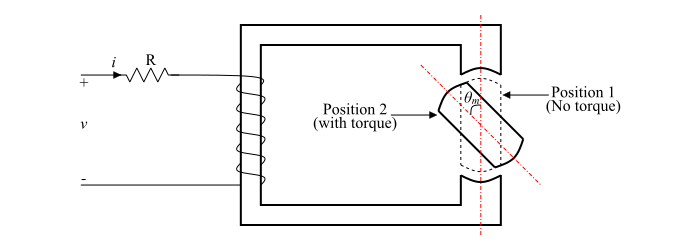# What is a Singly-Excited System? How to Calculate its Electromagnetic Torque?

A singly-excited system is type of excitation system used in electromechanical energy conversion which requires only one coil to produce the magnetic field. In the singly-excited system, there is only one set of electrical input terminals and one set of mechanical output terminals. The examples of singly-excited system are electromagnetic relay, hysteresis motor, solenoid valve etc.In a singly excited system, a coil is wound around a magnetic core and is connected to a voltage source so that it produces a magnetic field. Due to this magnetic field, the rotor which is made up of ferromagnetic material, experiences a torque urging it towards a region where the magnetic field is stronger, i.e., the torque exerted on the rotor tries to position it such that it gives minimum reluctance for the magnetic field. The reluctance depends upon the rotor angle. This torque is known as reluctance torque or saliency torque.

In order to analyse a singly-excited system, following assumptions are made −

• For any rotor position the relationship between flux linkage (ψ) and current is linear.

• The coil has negligible leakage flux.

• Hysteresis and eddy-current losses are neglected.

• All the electric fields are neglected and the magnetic field is predominating.

Let R is the resistance of the coil circuit. By applying KVL, the voltage equation for the circuit can be written as

$$\mathrm{v=iR+\frac{d\psi}{dt}\:\:\:\:\:\:...(1)}$$

Multiplying the eq. (1) by i, we get,

$$\mathrm{vi=i^{2}R+i\frac{d\psi}{dt}\:\:\:\:\:\:...(2)}$$

Integrating on both sides of eq. (2) and assuming that the initial condition being zero.

$$\mathrm{\int_{0}^{t}vi\:dt=\int_{0}^{t}i^2Rdt+\int_{0}^{t}i\frac{d\psi}{dt}dt}$$

$$\mathrm{\int_{0}^{t}vi\:dt=\int_{0}^{t}i^2Rdt+\int_{0}^{t}i\:d\psi\:\:\:\:\:\:...(3)}$$

The eq. (3) shows that the total electrical energy input is equal to the sum of two parts. The first is energy to electrical losses and the second is useful electrical energy, i.e.

$$\mathrm{[Total \:Electrical \:Energy\: Input\: W_{e}] = [Energy\: to\: Electrical\: Losses \:W_{electric.losses}]+[Useful \:Electrical \:Energy\: W_{f}+W_{m}]}$$

$$\mathrm{\Longrightarrow W_{e}=W_{electric.losses}+[W_{f}+W_{m}]\:\:\:\:\:\:...(4)}$$

And,

$$\mathrm{\int_{0}^{\psi}i\:d\psi=W_{f}+W_{m}\:\:\:\:\:\:...(5)}$$

## Stored Energy in the Magnetic Field

The instantaneous value of energy stored in the magnetic field depends upon the inductance and current values at that instant. For any stationary position of the rotor, the mechanical output is zero and all the useful electrical energy input is stored in the magnetic field.

$$\mathrm{W_{f}=\int_{0}^{\psi}i\:d\psi=\int_{0}^{\psi}\frac{\psi}{L}d\psi=\frac{\psi^2}{2L}\:\:\:\:\:\:...(6)}$$

For a magnetically linear system, ψ = Li, thus,

$$\mathrm{W_{f}=\frac{\psi^2}{2L}=\frac{1}{2}Li^2\:\:\:\:\:\:...(7)}$$

## Electromagnetic Torque

For a movement of the rotor (rotor angle $θ_{m}$), the energy to mechanical work corresponds to the loss of stored energy in the magnetic field. Therefore, the electromagnetic torque is,

$$\mathrm{\tau_{e}=\lim_{\Delta \theta \to 0}\lbrace-\frac{\Delta W_{f}}{\Delta\theta _{m}}\rbrace_{\psi =constant}}$$

$$\mathrm{\longrightarrow\tau_{e}=\lbrace-\frac{\partial W_{f}}{\partial\theta _{m}}\rbrace_{\psi =constant}=\lbrace-\frac{\partial}{\partial\theta_{m}}\frac{\psi^2}{2L}\rbrace_{\psi =constant}}$$

$$\mathrm{\Longrightarrow\tau_{e}=\frac{\psi^2}{L}\frac{1}{L^2}\frac{\partial L}{\partial\theta_{m}}=\frac{i^2}{2}\frac{\partial L}{\partial\theta_{m}}\:\:\:\:\:\:...(8)}$$

For a magnetically linear system, the electromagnetic torque is given by,

$$\mathrm{\tau_{e}=\frac{i^2}{2}\frac{dL}{d\theta_{m}}\:\:\:\:\:\:...(9)}$$